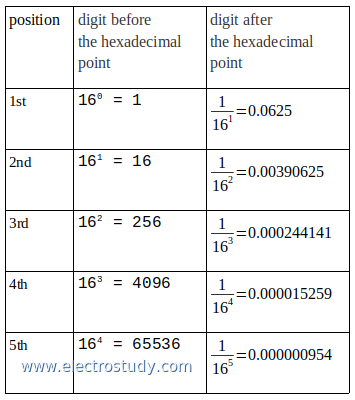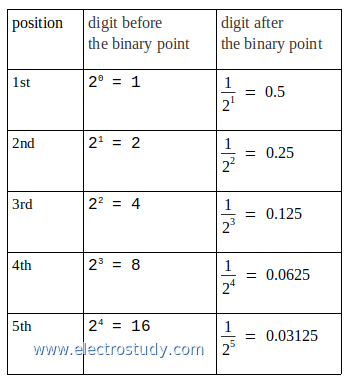## Table of the electric currents of a 3-phase motor

An electric motor has specifications: voltage is 380 V, 3 phase, frequency is 50 Hz, 1500 rpm, cos phi is 0.8 and real power on the name plate is 22 kW. Convert to HP (Horse Power) and calculate, how many current of electricity will flow into the motor?Connection of 3-Phase Motor

`1HP = 0.75 kW` --> 22 kW / 0.75 kW x 1 HP = 29.33 HP
--> 22 kW ≈ 30 HP
:catat: The power of the motor is 30 HP

V = 380 V 3 fase
f = 50 Hz
Cos φ = 0.8
P = 22 kW = 22000 W
I = ...?

The formula of real power electricity in 3-phase:
`P = √3 x V x I x Cos φ` --> I = P / (√3 x V x Cos φ)
--> I = 22000 / (√3 x 380 x 0.8)
--> I = 41.78 A
:catat: The current of electricity will flow into the motor is 41,78 A

For reference, here is a table of the electric currents of a 3-phase motor, 380 V, cos phi 0.8

kWHPA
0.40.50.8
0.7511.4
1.11.52.1
1.522.8
2.234.2
345.7
3.757
4.568.5
5.57.510.4
7.51014.2
91217.1
111520.9
152028.5
18.52535.1
223041.8
304057
375070.3
456085.5
5575104.5
75100142.4

## Hexadecimal fractions to decimal numbers

This post is explain how to convert hexadecimal fractions to decimal numbers. As we known, hexadecimal number is system number who have 16 digit numbers, which is 0, 1, 2, 3, 4, 5, 6, 7, 8, 9, A, B, C, D, E and F, where:
```A present of 10 B present of 11 C present of 12 D present of 13 E present of 14 F present of 15.```
The picture below is show hexadecimal fraction to decimal, form 1st position to 5th position of the digit, before and after the hexadecimal point.Hexadecimal fractions to decimal
Example 1:
Convert hexadecimal number 0.59A to decimal number

Completion:
0.59A (16) = ... (10)
1st digit after hexadecimal point: 5 → 5 × (1/ 161) = 5 × 0.0625 = 0.3125
2nd digit after hexadecimal point: 9 → 9 × (1/ 162) = 9 × 0.00390625 = 0.03515625
3rd digit after hexadecimal point: A → 10 × (1/ 163) = 10 × 0.000244141 = 0.00244141
sum all → 0.3125 + 0.03515625 + 0.00244141 = 0.35009766

0.59A (16) = 0.35009766 (10)

Example 2:
Convert hexadecimal number E2.81AC to decimal number

Completion:
E2.81AC (16) = ... (10)

1st digit: 2 → 2 × 160 = 2 × 1 = 2
2nd digit: E → 14 × 161 = 14 × 16 = 224
sum all → 2 + 224 = 226

1st digit: 8 → 8 × (1/ 161) = 8 × 0.0625 = 0.5
2nd digit: 1 → 1 × (1/ 162) = 1 × 0.00390625 = 0.00390625
3rd digit: A → 10 × (1/ 163) = 10 × 0.000244141 = 0.00244141
4th digit: C → 12 × (1/ 164) = 12 × 0.000015259 = 0.000183108
sum all → 0.5 + 0.00390625 + 0.00244141 + 0.000183108 = 0.506530768

E2.81AC (16) = 226.506530768 (10)

## Octal fractions to decimal numbers

This post is explain how to convert octal fractions to decimal numbers. The picture below is show octal fraction to decimal, form 1st position to 5th position of the digit, before and after the octal point.Octal fractions to decimal
Example 1:
Convert Octal number 0.725 to decimal number

Completion:
0.725 (8) = ... (10)
1st digit after octal point: 7 → 7 × (1/ 81) = 7 × 0.125 = 0.875
2nd digit after octal point: 2 → 2 × (1/ 82) = 2 × 0.015625 = 0.03125
3rd digit after octal point: 5 → 5 × (1/ 83) = 5 × 0.001953125 = 0.009765625
sum all → 0.875 + 0.03125 + 0.009765625 = 0.916015625

0.725 (8) = 0.916015625 (10)

Example 2:
Convert octal number 105.2063 to decimal number

Completion:
105.2063 (8) = ... (10)

before octal point
1st digit: 5 → 5 × 80 = 5 × 1 = 5
2nd digit: 0 → 0 × 81 = 0 × 8 = 0
3rd digit: 1 → 1 × 82 = 1 × 64 = 64
sum all → 5 + 0 + 64 = 69

after octal point
1st digit: 2 → 2 × (1/ 81) = 2 × 0.125 = 0.25
2nd digit: 0 → 0 × (1/ 82) = 0 × 0.015625 = 0
3rd digit: 6 → 6 × (1/ 83) = 6 × 0.001953125 = 0.01171875
4th digit: 3 → 3 × (1/ 84) = 3 × 0.000244141 = 0.000732423
sum all → 0.25 + 0 + 0.01171875 + 0.000732423 = 0.262451173

501.2063 (8) = 69.262451173 (10)

## Binary fractions to decimal numbers

This post is explain how to convert binary fractions to decimal numbers. The picture below is show binary fraction to decimal, form 1st position to 5th position of the digit, before and after the binary point.Binary fractions to decimal
Example 1:
Convert binary number 0.1101 to decimal number

Completion:
0.101 (2) = ... (10)
1st digit after binary point: 1 → 1 × (1/ 21) = 1 × 0.5 = 0.5
2nd digit after binary point: 0 → 0 × (1/ 22) = 0 × 0.25 = 0
3rd digit after binary point: 1 → 1 × (1/ 23) = 1 × 0.125 = 0.125
sum all → 0.5 + 0 + 0.125 = 0.625

0.101 (2) = 0.625 (10)

Example 2:
Convert binary number 1110.11011 to decimal number

Completion:
1110.11011 (2) = ... (10)

before binary point
1st digit: 0 → 1 × 20 = 0 × 1 = 0
2nd digit: 1 → 1 × 21 = 1 × 2 = 2
3rd digit: 1 → 1 × 22 = 1 × 4 = 4
4th digit: 1 → 1 × 23 = 1 × 8 = 8
sum all → 0 + 2 + 4 + 8 = 14

after binary point
1st digit: 1 → 1 × (1/ 21) = 1 × 0.5 = 0.5
2nd digit: 1 → 1 × (1/ 22) = 1 × 0.25 = 0.25
3rd digit: 0 → 0 × (1/ 23) = 0 × 0.125 = 0
4th digit: 1 → 1 × (1/ 24) = 1 × 0.0625 = 0.0625
5th digit: 1 → 1 × (1/ 25) = 1 × 0.03125 = 0.03125
sum all → 0.5 + 0.25 + 0 + 0.0625 + 0.03125 = 0.84375

1110.11011 (2) = 14.84375 (10)